/

### The gamma distribution

The gamma distribution is a two-parameter family of continuous probability distributions. Two different parameterisations are in common use, see below, with the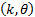parameterisation being apparently somewhat more common in econometrics and the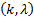parameterisation being somewhat more common in Bayesian statistics.

 Distribution name Gamma distribution Common notation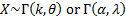Parameters Has two commonly used parameterisations: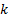= shape parameter (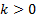)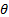= scale parameter (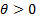) or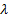= inverse scale (i.e. rate) parameter () where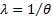. Unless otherwise specified the material below assumes the first parameterisation (i.e. using a scale parameter) Domain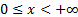Probability density function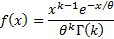Cumulative distribution function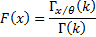Mean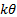Variance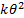Skewness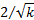(Excess) kurtosis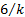Moment generating function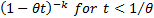Characteristic function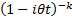Other comments The gamma distribution can also be defined with a location parameter,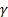, say, in which case its domain is shifted to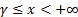.   Its mode is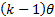for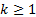.   If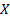follows an exponential distribution with rate parameterthen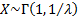.   Iffollows a chi-squared distribution, with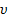degrees of freedom, i.e. i.e.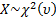then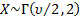and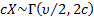.   Ifis integral then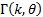is also called the Erlang distribution. It is the distribution of the sum ofindependent exponential variables each with mean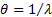. Events that occur independently with some average rate are commonly modelled using a Poisson process. The waiting times betweenoccurrences of the event are then Erlang distributed whilst the number of events in a given amount of time is Poisson distributed.   Iffollows a Maxwell-Boltzmann distribution with parameter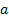then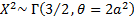. Iffollows a skew logistic distribution with parameterthen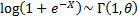.   The gamma distribution is the conjugate prior for the precision (i.e. inverse variance) of a normal distribution and for the exponential distribution.   The gamma distribution has the ‘summation’ property that if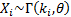for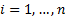and the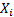are independent then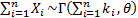.   Its non-central moments (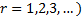are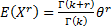. There is in general no simple closed form for its median.

Nematrian web functions

Functions relating to the above distribution may be accessed via the Nematrian web function library by using a DistributionName of “gamma”. Functions relating to a generalised version of this distribution including an additional location (i.e. shift) parameter may be accessed by using a DistributionName of “gamma3”, see also including additional shift and scale parameters. For details of other supported probability distributions see here.